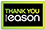25% off almost everything | use code: FLASHSALE | Hurry, Ends at midnight!

# Numerical approximation of partial differential equations

Category: Mathematics
Finite element methods for approximating partial differential equations have reached a high degree of maturity, and are an indispensible tool in science and technology. This textbook aims at providing a thorough introduction to the construction, analysis, and implementation of finite element methods for model problems arising in continuum mechanics. The first part of the book discusses elementary properties of linear partial differential equations along with their basic numerical approximation, the functional-analytical framework for rigorously establishing existence of solutions, and the construction and analysis of basic finite element methods. The second part is devoted to the optimal adaptive approximation of singularities and the fast iterative solution of linear systems of equations arising from finite element discretizations. In the third part, the mathematical framework for analyzing and discretizing saddle-point problems is formulated, corresponding finte element methods are analyzed, and particular applications including incompressible elasticity, thin elastic objects, electromagnetism, and fluid mechanics are addressed. The book includes theoretical problems and practical projects for all chapters, and an introduction to the implementation of finite element methods.
€100.67302 Reward Points
In stock online
Delivery in 5 - 7 working days

Any purchases for more than €10 are eligible for free delivery anywhere in the UK or Ireland!

Finite element methods for approximating partial differential equations have reached a high degree of maturity, and are an indispensible tool in science and technology. This textbook aims at providing a thorough introduction to the construction, analysis, and implementation of finite element methods for model problems arising in continuum mechanics. The first part of the book discusses elementary properties of linear partial differential equations along with their basic numerical approximation, the functional-analytical framework for rigorously establishing existence of solutions, and the construction and analysis of basic finite element methods. The second part is devoted to the optimal adaptive approximation of singularities and the fast iterative solution of linear systems of equations arising from finite element discretizations. In the third part, the mathematical framework for analyzing and discretizing saddle-point problems is formulated, corresponding finte element methods are analyzed, and particular applications including incompressible elasticity, thin elastic objects, electromagnetism, and fluid mechanics are addressed. The book includes theoretical problems and practical projects for all chapters, and an introduction to the implementation of finite element methods.
Quantity:
In stock online
Delivery in 5 - 7 working days302 Reward Points

Any purchases for more than €10 are eligible for free delivery anywhere in the UK or Ireland!

€100.67
In stock online
Delivery in 5 - 7 working days
Quantity:302 Reward Points

Any purchases for more than €10 are eligible for free delivery anywhere in the UK or Ireland!

### Product Description

Finite element methods for approximating partial differential equations have reached a high degree of maturity, and are an indispensible tool in science and technology. This textbook aims at providing a thorough introduction to the construction, analysis, and implementation of finite element methods for model problems arising in continuum mechanics. The first part of the book discusses elementary properties of linear partial differential equations along with their basic numerical approximation, the functional-analytical framework for rigorously establishing existence of solutions, and the construction and analysis of basic finite element methods. The second part is devoted to the optimal adaptive approximation of singularities and the fast iterative solution of linear systems of equations arising from finite element discretizations. In the third part, the mathematical framework for analyzing and discretizing saddle-point problems is formulated, corresponding finte element methods are analyzed, and particular applications including incompressible elasticity, thin elastic objects, electromagnetism, and fluid mechanics are addressed. The book includes theoretical problems and practical projects for all chapters, and an introduction to the implementation of finite element methods.

### Product Details

#### Numerical approximation of partial differential equations

ISBN9783319323534

Format

PublisherSPRINGER (09 June. 2016)

No. of Pages535

Weight9457

Language English (United States)

Dimensions 235 x 155 x 36